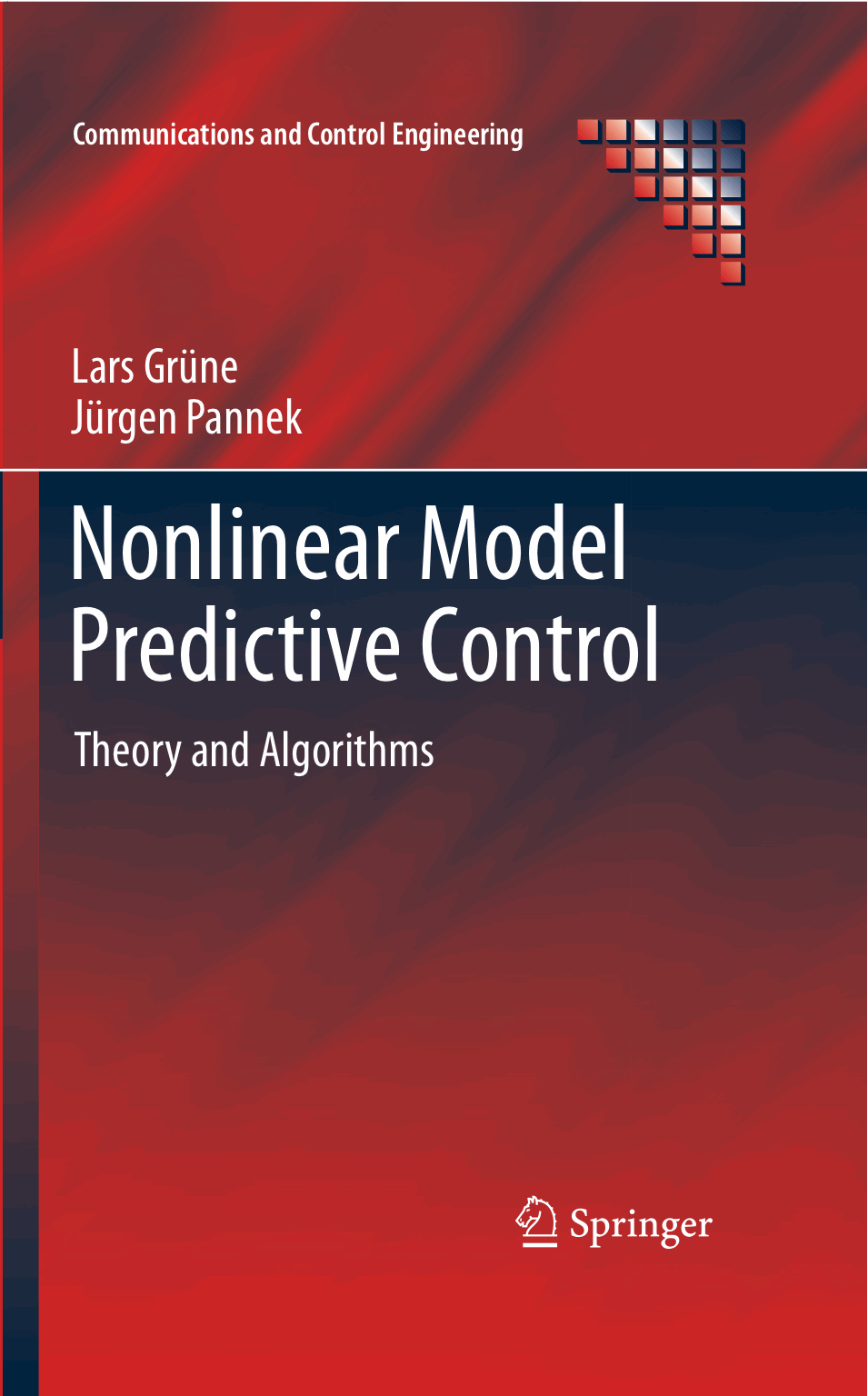## Errata for the 1st edition

Here you can find corrections for those errors in the first edition of the book that we are aware of:

• page 32, line -5:   replace "x \in X" by "x \in S" in the definition of \widetilde S

• page 40, Problem 2(a):   the difference equation must read x(n+1) = 1/2 x(n) + sin(n+1)

• page 40, Problem 2(c):   the difference equation must read x(n+1) = (n+1)/(n+2) x(n) + sin(n+1)

• page 49, Definition 3.6:   replace "r_g, r_h" by "p_g, p_h"

• page 54, 5th line after (3):   replace "\omega_{N_k}" by "\omega_{N-k}"

• page 56, line 5:   replace "a special cases" by "as special cases"

• page 61, line -6:   replace "x_0" by "x(k)" in the argument of V_{N-k}

• page 89, Formula (5.7):   replace "U(...)" by "IU(...)" (double strike U)

• page 94, Example 5.8:   replace "IR" by "IR^2" (two times after the formula containing x_u(0,x) etc.)

• page 104, line -2:   replace "k\in IN_0" by "n\in IN_0"

• page 108, line 7:   replace "(5.4)" by "(5.10) or (5.18), respectively"

• page 126, line -2f:   replace "only about" by "at most about"

• page 176, Formula (7.8):   Replace "V(...)" by "F(...)" (two times) on the left hand side

• page 230, line 13:   replace "\alpha \alpha_3(\sigma/2)/2" by "\alpha \alpha_3(\sigma)/2"

• page 239, Formula (8.15):   replace "V_N( f(x,\mu_N(x) + d) )" by "V_N( f(x,\mu_N(x)) + d )"

If you should discover further errors, we would appreciate a notification via email.

→ back to homepage www.nmpc-book.com

© 2011-2018 Lars Grüne, Jürgen Pannek, errata last updated on 28th April 2018